Скачать презентацию Chapter 22 Electromagnetism Known since antiquity

• Количество слайдов: 33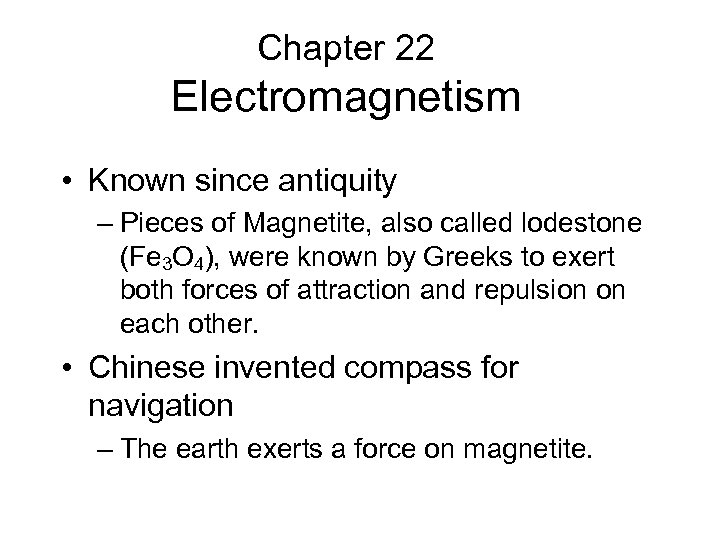Chapter 22 Electromagnetism • Known since antiquity – Pieces of Magnetite, also called lodestone (Fe 3 O 4), were known by Greeks to exert both forces of attraction and repulsion on each other. • Chinese invented compass for navigation – The earth exerts a force on magnetite.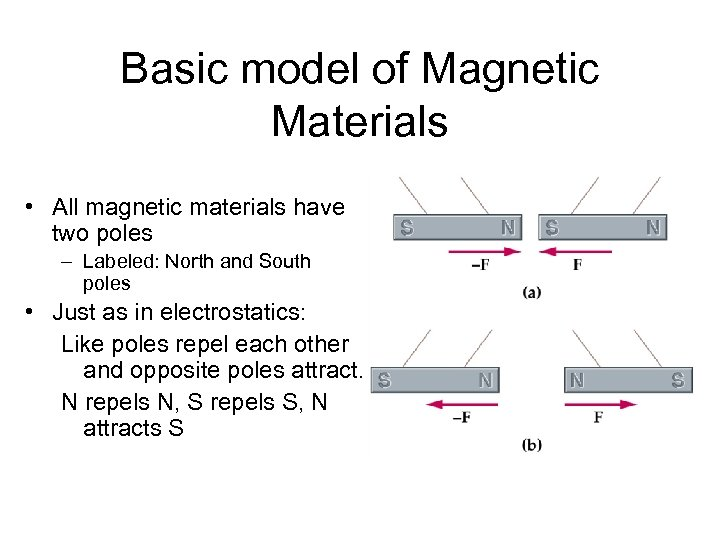Basic model of Magnetic Materials • All magnetic materials have two poles – Labeled: North and South poles • Just as in electrostatics: Like poles repel each other and opposite poles attract. N repels N, S repels S, N attracts S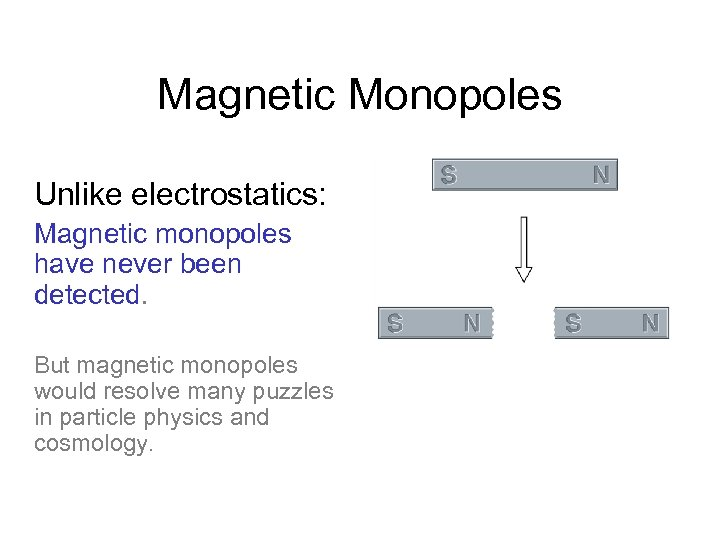Magnetic Monopoles Unlike electrostatics: Magnetic monopoles have never been detected. But magnetic monopoles would resolve many puzzles in particle physics and cosmology.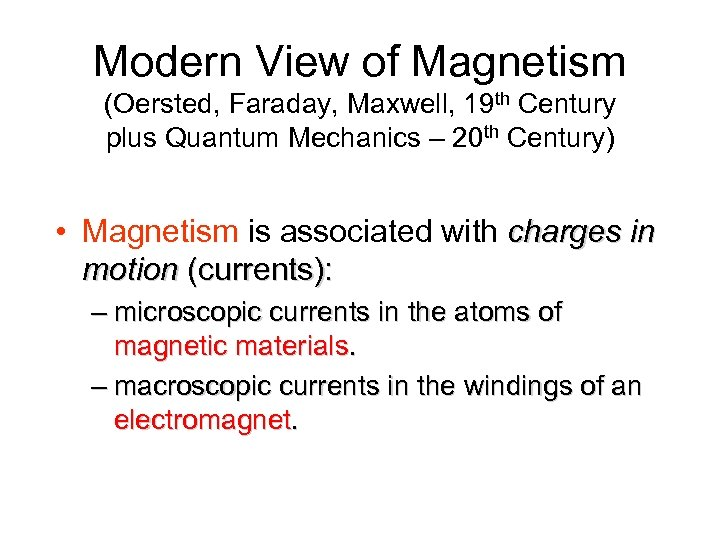Modern View of Magnetism (Oersted, Faraday, Maxwell, 19 th Century plus Quantum Mechanics – 20 th Century) • Magnetism is associated with charges in motion (currents): – microscopic currents in the atoms of magnetic materials. – macroscopic currents in the windings of an electromagnet.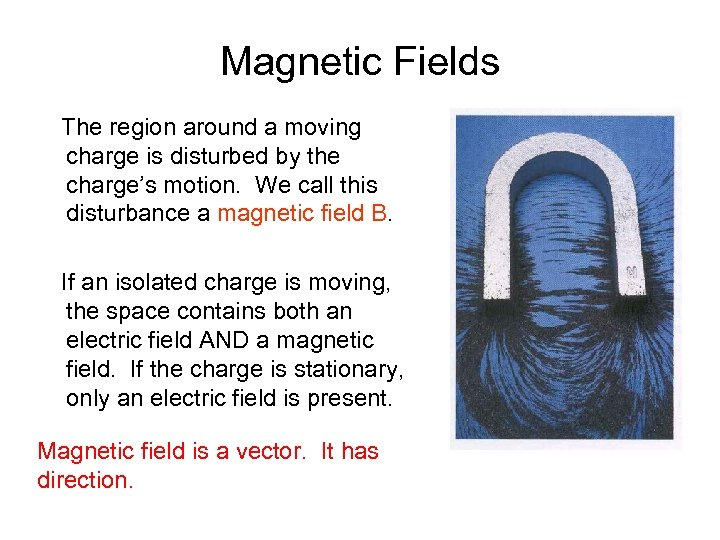Magnetic Fields The region around a moving charge is disturbed by the charge’s motion. We call this disturbance a magnetic field B. If an isolated charge is moving, the space contains both an electric field AND a magnetic field. If the charge is stationary, only an electric field is present. Magnetic field is a vector. It has direction.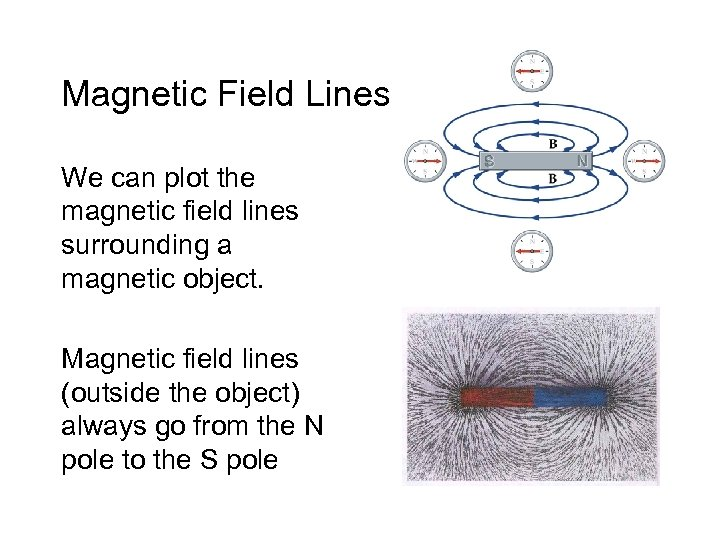Magnetic Field Lines We can plot the magnetic field lines surrounding a magnetic object. Magnetic field lines (outside the object) always go from the N pole to the S pole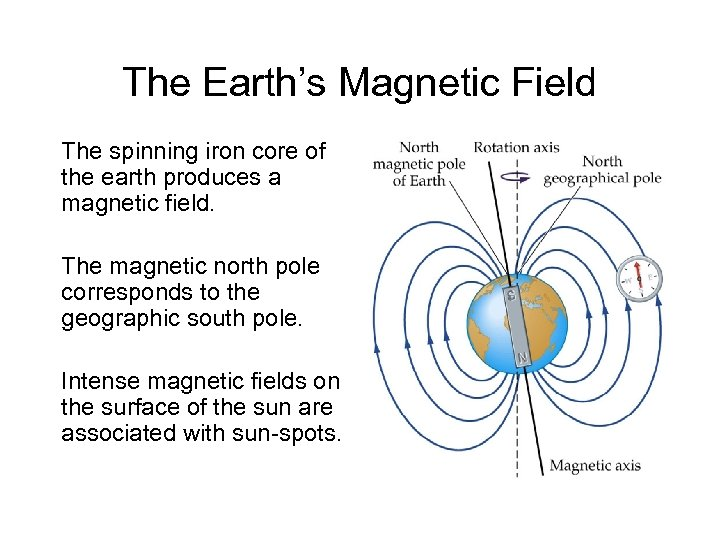The Earth’s Magnetic Field The spinning iron core of the earth produces a magnetic field. The magnetic north pole corresponds to the geographic south pole. Intense magnetic fields on the surface of the sun are associated with sun-spots.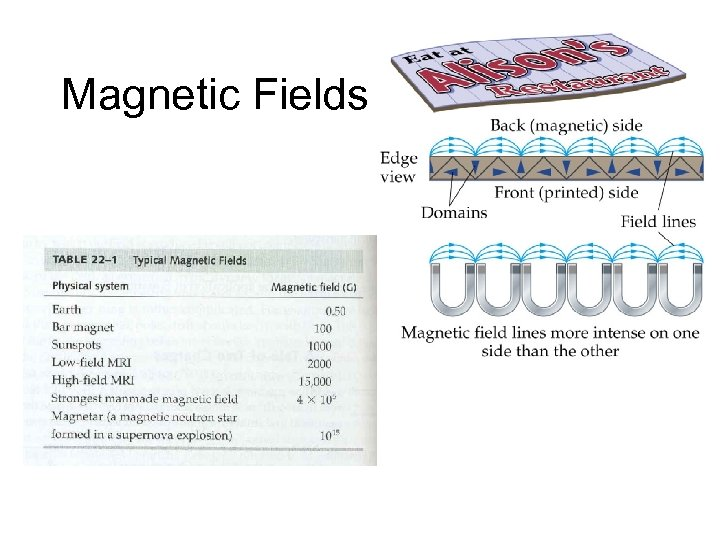Magnetic Fields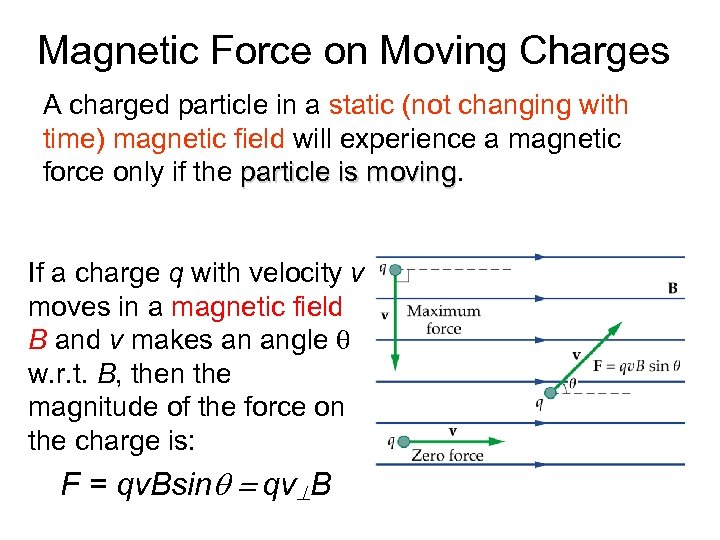Magnetic Force on Moving Charges A charged particle in a static (not changing with time) magnetic field will experience a magnetic force only if the particle is moving If a charge q with velocity v moves in a magnetic field B and v makes an angle q w. r. t. B, then the magnitude of the force on the charge is: F = qv. Bsinq = qv B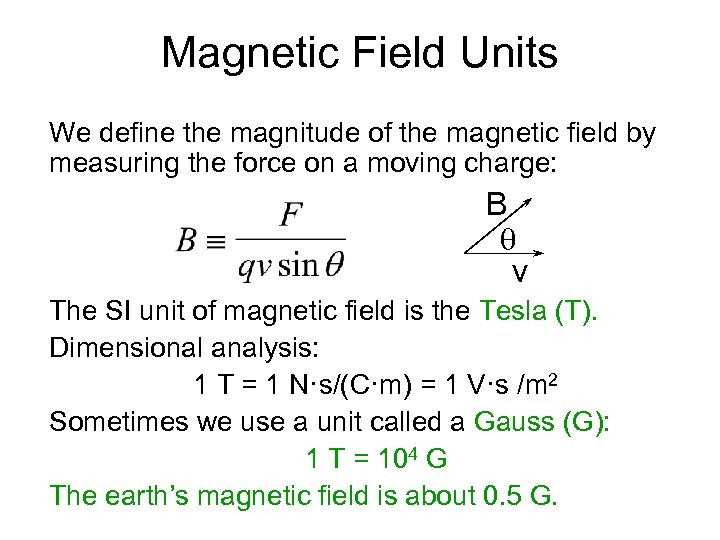Magnetic Field Units We define the magnitude of the magnetic field by measuring the force on a moving charge: B q v The SI unit of magnetic field is the Tesla (T). Dimensional analysis: 1 T = 1 N·s/(C·m) = 1 V·s /m 2 Sometimes we use a unit called a Gauss (G): 1 T = 104 G The earth’s magnetic field is about 0. 5 G.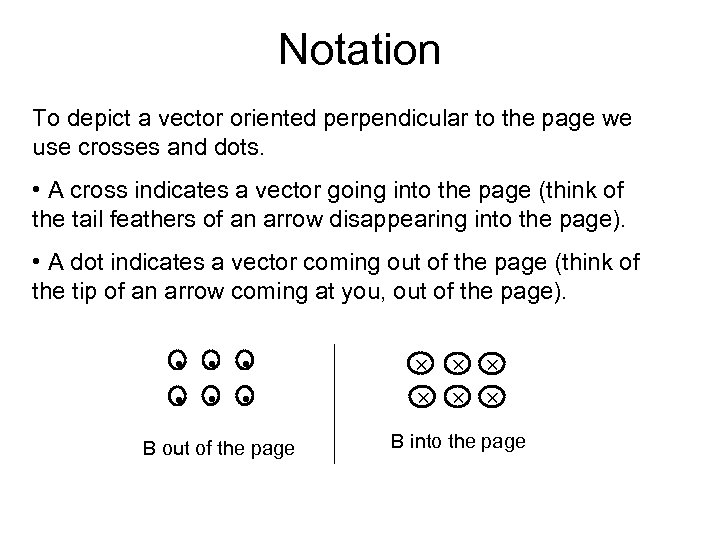Notation To depict a vector oriented perpendicular to the page we use crosses and dots. • A cross indicates a vector going into the page (think of the tail feathers of an arrow disappearing into the page). • A dot indicates a vector coming out of the page (think of the tip of an arrow coming at you, out of the page). • • • B out of the page B into the page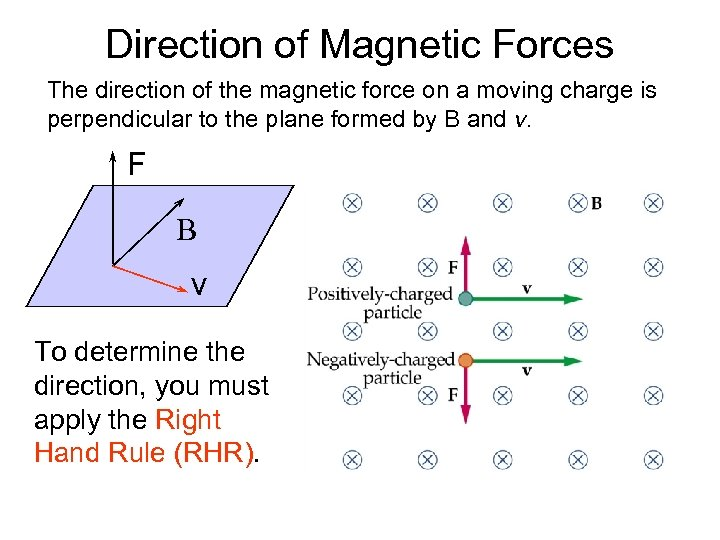Direction of Magnetic Forces The direction of the magnetic force on a moving charge is perpendicular to the plane formed by B and v. F B v To determine the direction, you must apply the Right Hand Rule (RHR).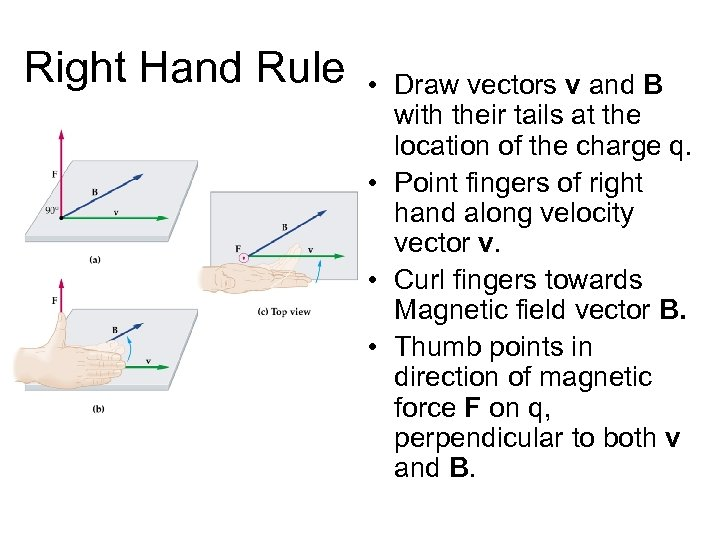Right Hand Rule • Draw vectors v and B with their tails at the location of the charge q. • Point fingers of right hand along velocity vector v. • Curl fingers towards Magnetic field vector B. • Thumb points in direction of magnetic force F on q, perpendicular to both v and B.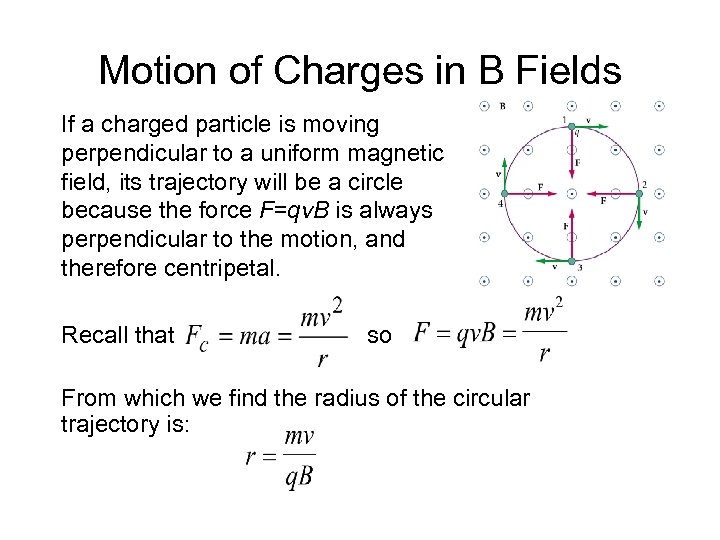Motion of Charges in B Fields If a charged particle is moving perpendicular to a uniform magnetic field, its trajectory will be a circle because the force F=qv. B is always perpendicular to the motion, and therefore centripetal. Recall that so From which we find the radius of the circular trajectory is: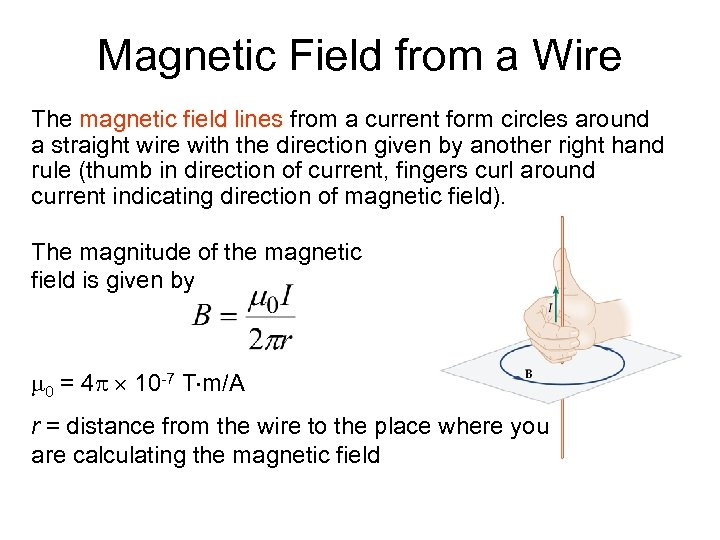Magnetic Field from a Wire The magnetic field lines from a current form circles around a straight wire with the direction given by another right hand rule (thumb in direction of current, fingers curl around current indicating direction of magnetic field). The magnitude of the magnetic field is given by m 0 = 4 p 10 -7 T m/A r = distance from the wire to the place where you are calculating the magnetic field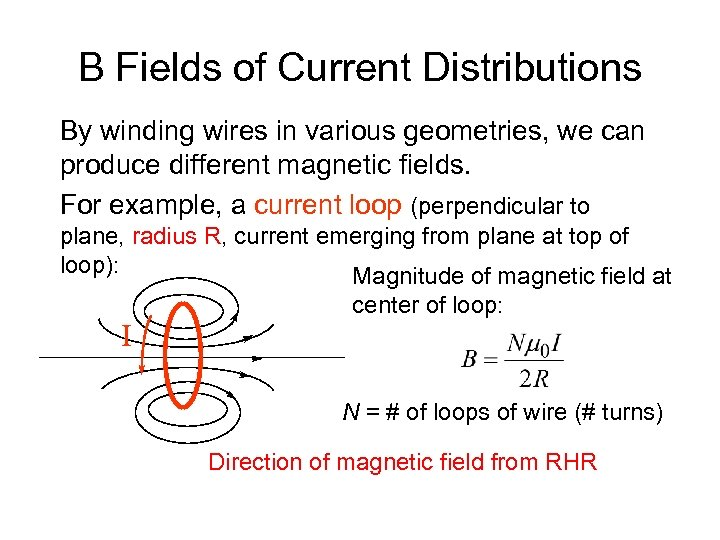B Fields of Current Distributions By winding wires in various geometries, we can produce different magnetic fields. For example, a current loop (perpendicular to plane, radius R, current emerging from plane at top of loop): Magnitude of magnetic field at I center of loop: N = # of loops of wire (# turns) Direction of magnetic field from RHR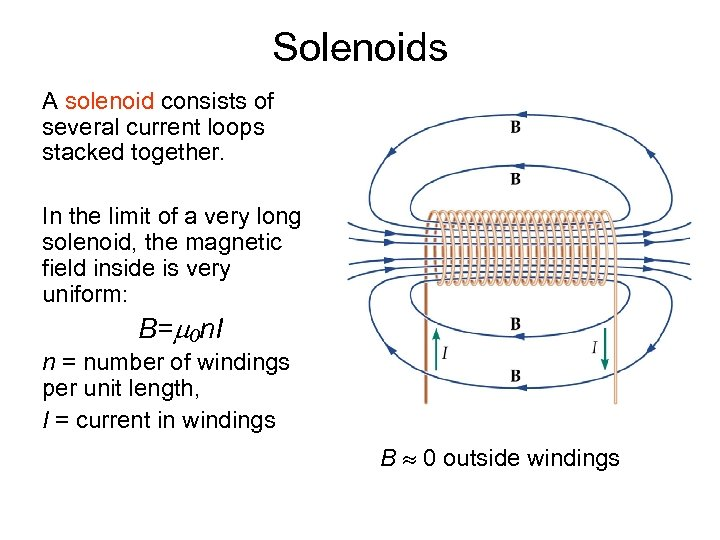Solenoids A solenoid consists of several current loops stacked together. In the limit of a very long solenoid, the magnetic field inside is very uniform: B=m 0 n. I n = number of windings per unit length, I = current in windings B 0 outside windings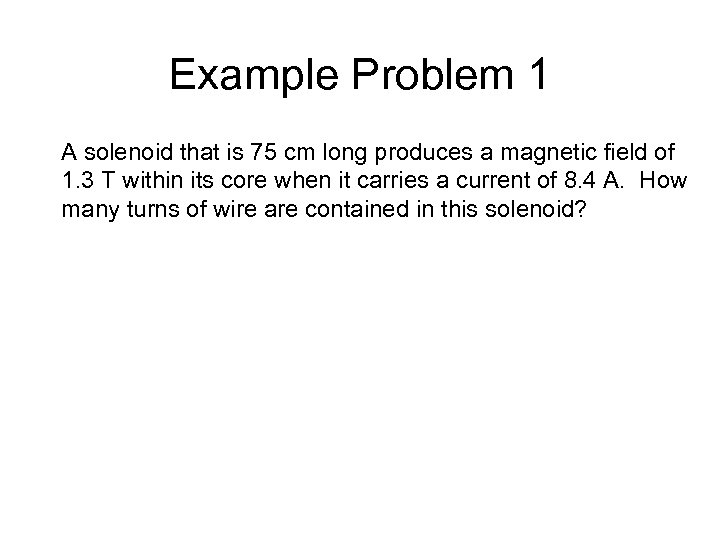Example Problem 1 A solenoid that is 75 cm long produces a magnetic field of 1. 3 T within its core when it carries a current of 8. 4 A. How many turns of wire are contained in this solenoid?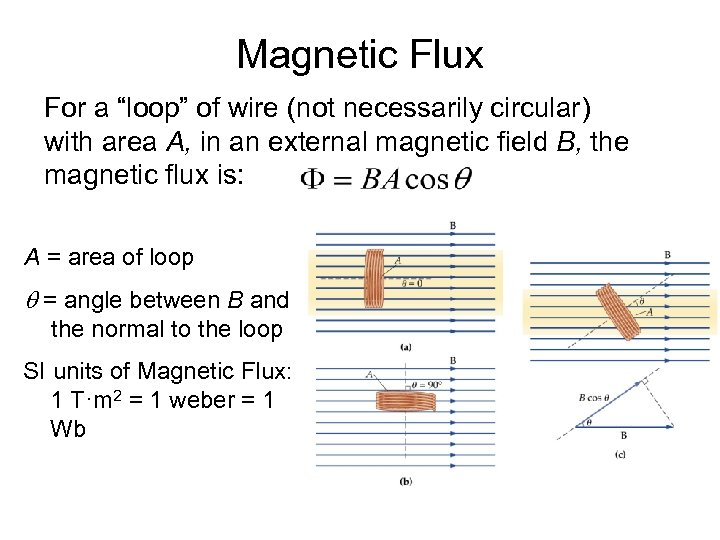Magnetic Flux For a “loop” of wire (not necessarily circular) with area A, in an external magnetic field B, the magnetic flux is: A = area of loop q = angle between B and the normal to the loop SI units of Magnetic Flux: 1 T·m 2 = 1 weber = 1 Wb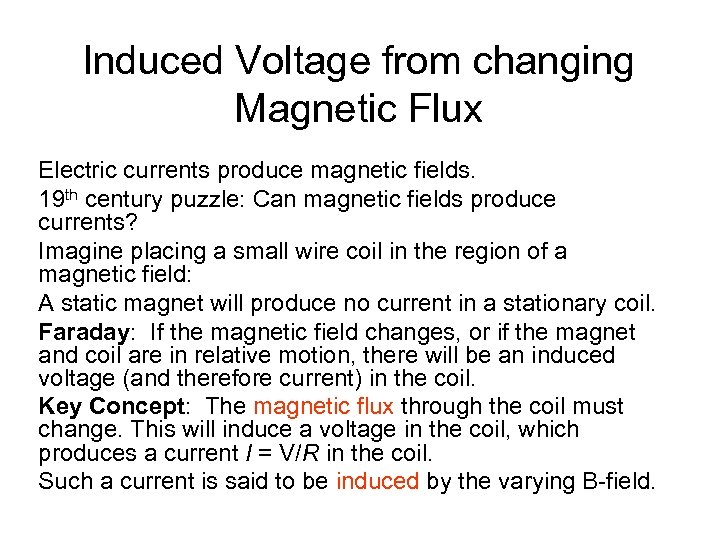Induced Voltage from changing Magnetic Flux Electric currents produce magnetic fields. 19 th century puzzle: Can magnetic fields produce currents? Imagine placing a small wire coil in the region of a magnetic field: A static magnet will produce no current in a stationary coil. Faraday: If the magnetic field changes, or if the magnet and coil are in relative motion, there will be an induced voltage (and therefore current) in the coil. Key Concept: The magnetic flux through the coil must change. This will induce a voltage in the coil, which produces a current I = V/R in the coil. Such a current is said to be induced by the varying B-field.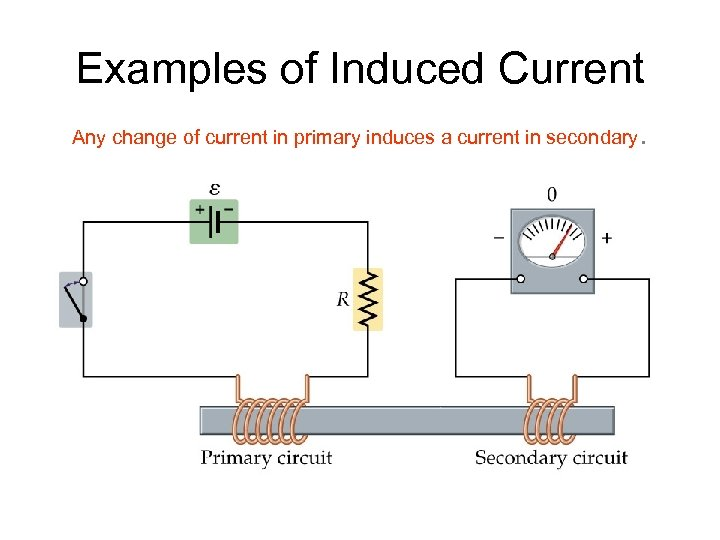Examples of Induced Current Any change of current in primary induces a current in secondary .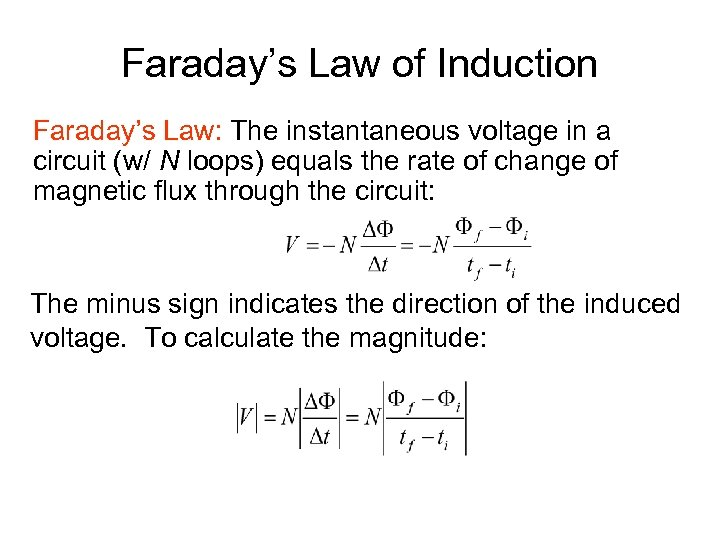Faraday’s Law of Induction Faraday’s Law: The instantaneous voltage in a circuit (w/ N loops) equals the rate of change of magnetic flux through the circuit: The minus sign indicates the direction of the induced voltage. To calculate the magnitude: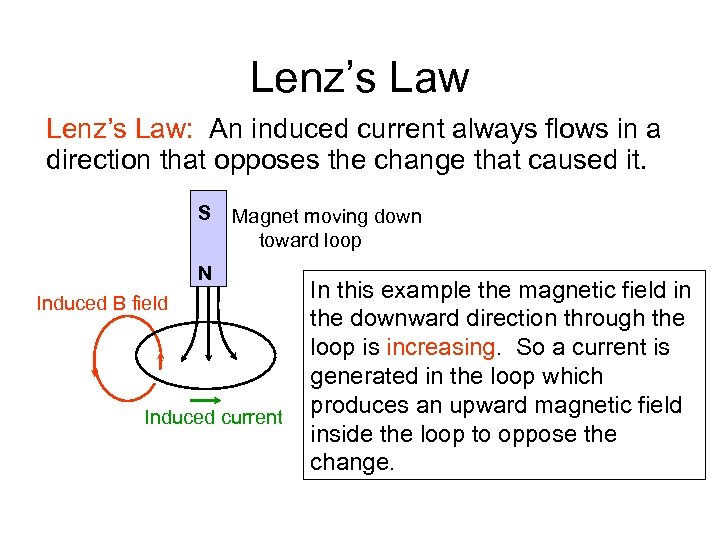Lenz’s Law: An induced current always flows in a direction that opposes the change that caused it. S Magnet moving down toward loop N Induced B field Induced current In this example the magnetic field in the downward direction through the loop is increasing. So a current is generated in the loop which produces an upward magnetic field inside the loop to oppose the change.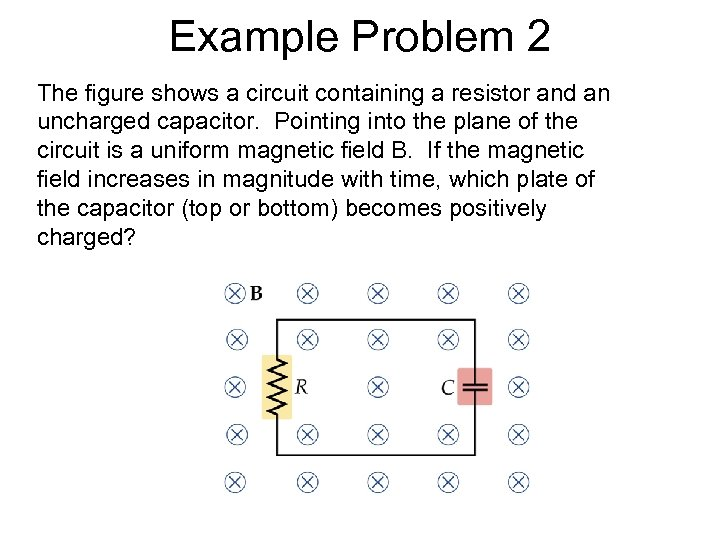Example Problem 2 The figure shows a circuit containing a resistor and an uncharged capacitor. Pointing into the plane of the circuit is a uniform magnetic field B. If the magnetic field increases in magnitude with time, which plate of the capacitor (top or bottom) becomes positively charged?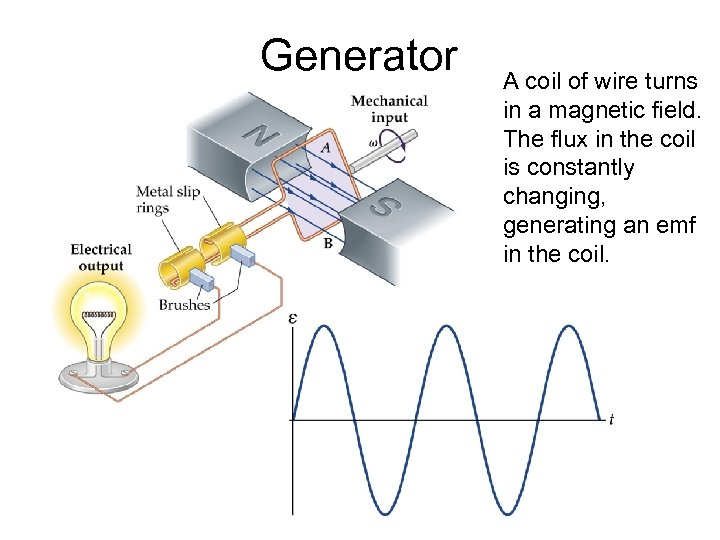Generator A coil of wire turns in a magnetic field. The flux in the coil is constantly changing, generating an emf in the coil.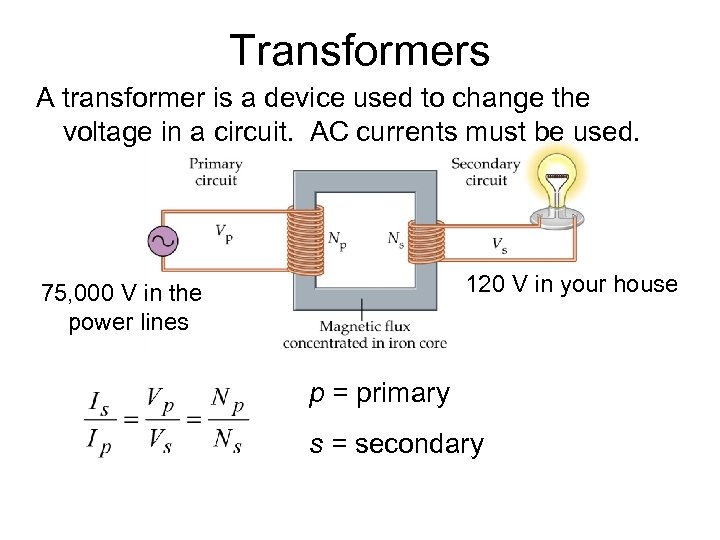Transformers A transformer is a device used to change the voltage in a circuit. AC currents must be used. 120 V in your house 75, 000 V in the power lines p = primary s = secondary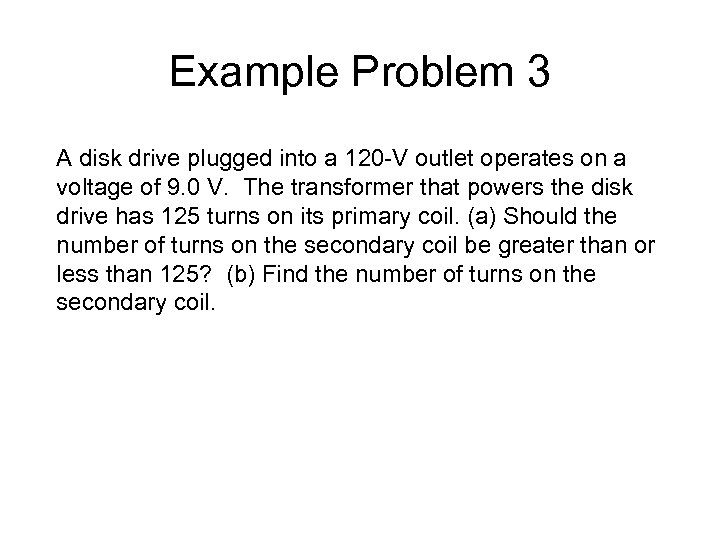Example Problem 3 A disk drive plugged into a 120 -V outlet operates on a voltage of 9. 0 V. The transformer that powers the disk drive has 125 turns on its primary coil. (a) Should the number of turns on the secondary coil be greater than or less than 125? (b) Find the number of turns on the secondary coil.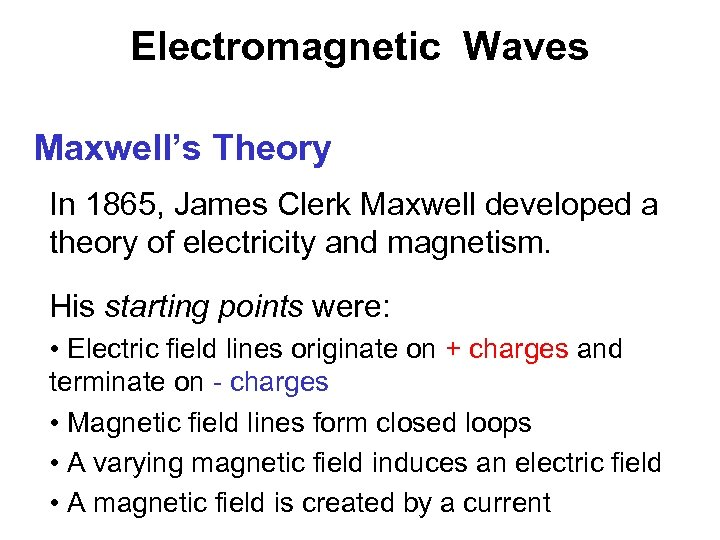Electromagnetic Waves Maxwell’s Theory In 1865, James Clerk Maxwell developed a theory of electricity and magnetism. His starting points were: • Electric field lines originate on + charges and terminate on - charges • Magnetic field lines form closed loops • A varying magnetic field induces an electric field • A magnetic field is created by a current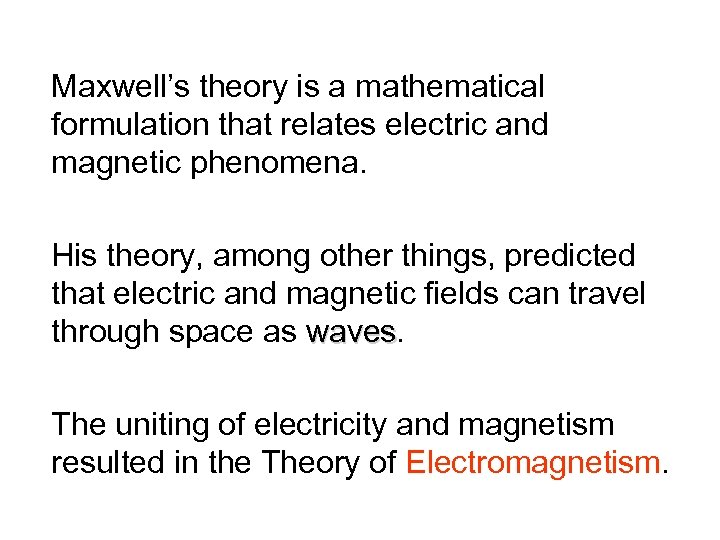Maxwell’s theory is a mathematical formulation that relates electric and magnetic phenomena. His theory, among other things, predicted that electric and magnetic fields can travel through space as waves The uniting of electricity and magnetism resulted in the Theory of Electromagnetism.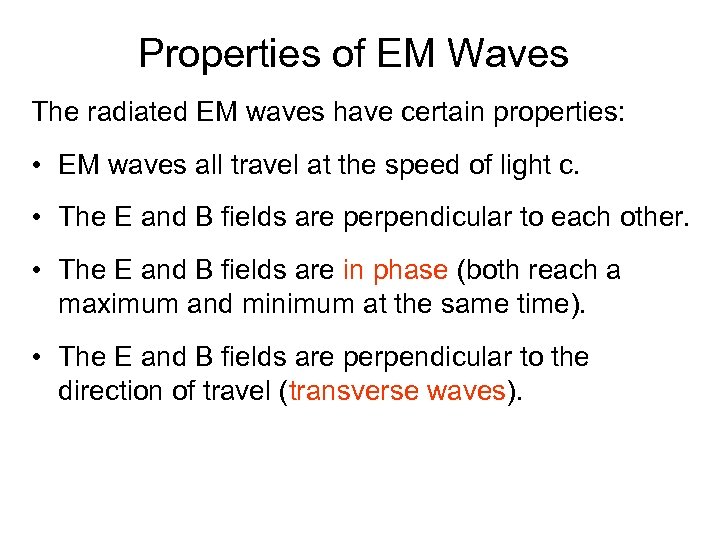Properties of EM Waves The radiated EM waves have certain properties: • EM waves all travel at the speed of light c. • The E and B fields are perpendicular to each other. • The E and B fields are in phase (both reach a maximum and minimum at the same time). • The E and B fields are perpendicular to the direction of travel (transverse waves).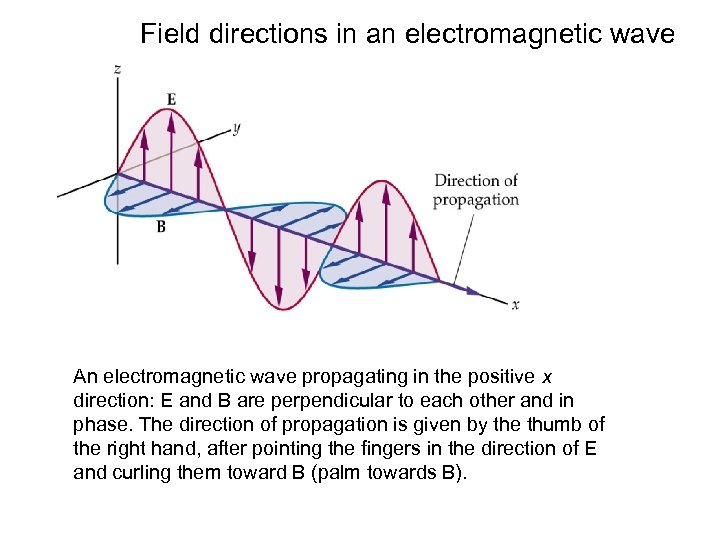Field directions in an electromagnetic wave An electromagnetic wave propagating in the positive x direction: E and B are perpendicular to each other and in phase. The direction of propagation is given by the thumb of the right hand, after pointing the fingers in the direction of E and curling them toward B (palm towards B).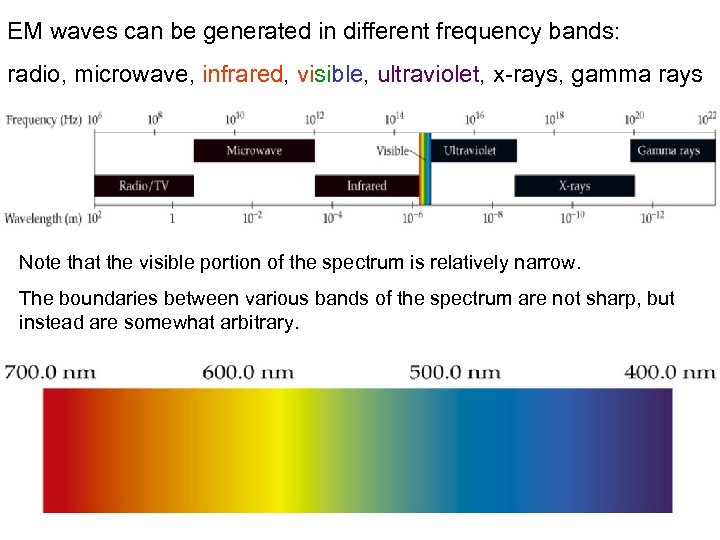EM waves can be generated in different frequency bands: radio, microwave, infrared, visible, ultraviolet, x-rays, gamma rays Note that the visible portion of the spectrum is relatively narrow. The boundaries between various bands of the spectrum are not sharp, but instead are somewhat arbitrary.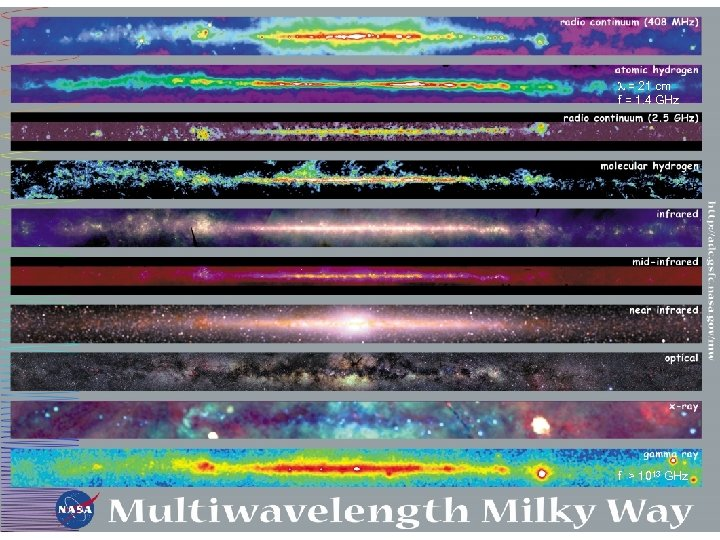l = 21 cm f = 1. 4 GHz f = 115 GHz f > 1013 GHz